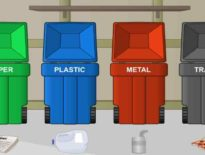# High School Math Activity: Create A Number Line

Use this number line to practice complex problems. Plot numbers, find the opposite, find the absolute value, and plot inequalities, or just use the tool to add and subtract. Set any value for the ‘x’ coordinates and then see if you can place the markers and lines in the correct places on the number line.

More high school math activities

This interactive activity is embedded in our High School Math course.

Students develop algebraic fluency by learning the skills needed to solve equations and perform manipulations with numbers, variables, equations, and inequalities. They also learn concepts central to the abstraction and generalization that algebra makes possible. Students learn to use number properties to simplify expressions or justify statements; describe sets with set notation and find the union and intersection of sets; simplify and evaluate expressions involving variables, fractions, exponents, and radicals; work with integers, rational numbers, and irrational numbers; and graph and solve equations, inequalities, and systems of equations. They learn to determine whether a relation is a function and how to describe its domain and range; use factoring, formulas, and other techniques to solve quadratic and other polynomial equations; formulate and evaluate valid mathematical arguments using various types of reasoning; and translate word problems into mathematical equations and then use the equations to solve the original problems.

Request a free catalog of K-12 Courses

Request the Scope & Sequence for a course

## High School Math Activity: Create A Number Line